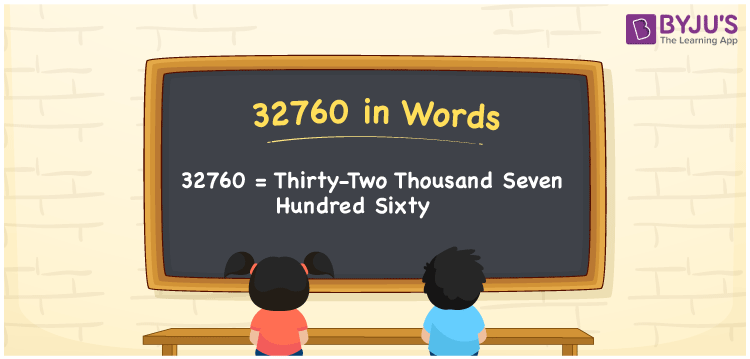# 32760 in Words

The number of 32760 is written as “thirty-two thousand seven hundred sixty”. To convert the number 32760 into words, we generally prefer the place value system. For example, if you spent Rs. 32760 on purchasing a two-wheeler, you can write it as “I spent Rs. Thirty-two thousand seven hundred sixty on purchasing a two-wheeler”. Also, 32760 is a cardinal number

 32760 in Words: Thirty-two Thousand Seven Hundred Sixty. Thirty-two Thousand Seven Hundred Sixty in Numerical Form: 32760.

## 32760 in English Words## How to Write 32760 in Words?

Go through the below table to learn the place values of the numeral 32760.

 Ten-thousands Thousands Hundreds Tens Ones 3 2 7 6 0

The expanded form of 32760 is as follows:

= 3 × Ten thousand + 2 × Thousand + 7 × Hundred + 6 × Ten + 0 × One

= 3 × 10000 + 2 × 1000 + 7 × 100 + 6 × 10 + 0 × 1

= 30000 + 2000 + 700 + 60

= 32760

= Thirty-two thousand seven hundred sixty

Hence, 32760 in words is thirty-two thousand seven hundred sixty.

32760 in words – Thirty-two thousand seven hundred sixty

Is 32760 an odd number? – No

Is 32760 an even number? – Yes

Is 32760 a perfect square number? – No

Is 32760 a perfect cube number? – No

Is 32760 a prime number? – No

Is 32760 a composite number? – Yes

## Frequently Asked Questions on 32760 in Words

Q1

### How to spell 32760 in words?

32760 in words is thirty-two thousand seven hundred sixty.

Q2

### Simplify 32000 + 760, and express it in words.

Simplifying 32000 + 760, we get 32760. Hence, 32760 in words is thirty-two thousand seven hundred sixty.

Q3

### Is 32760 an odd number?

No, 32760 is not an odd number.